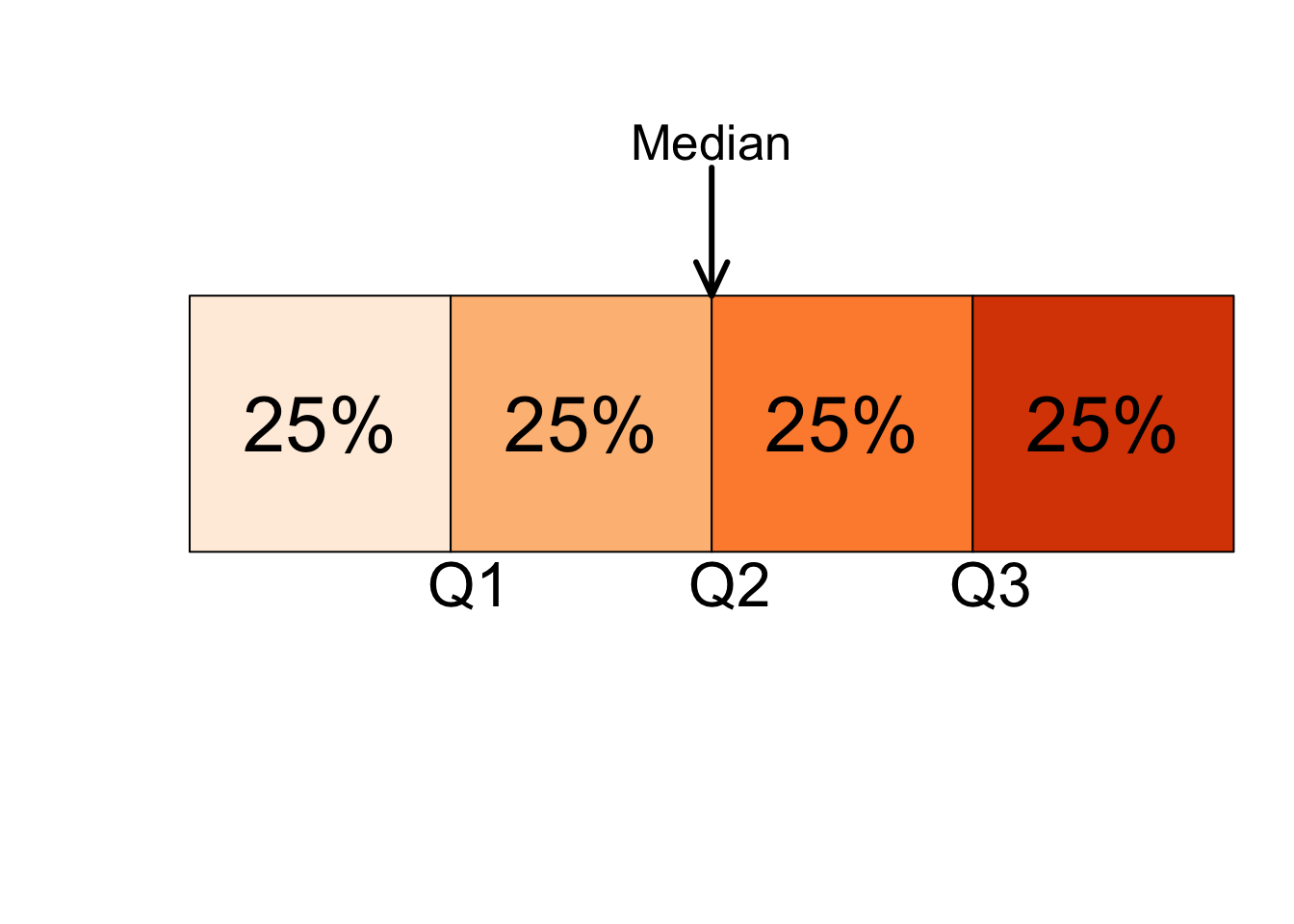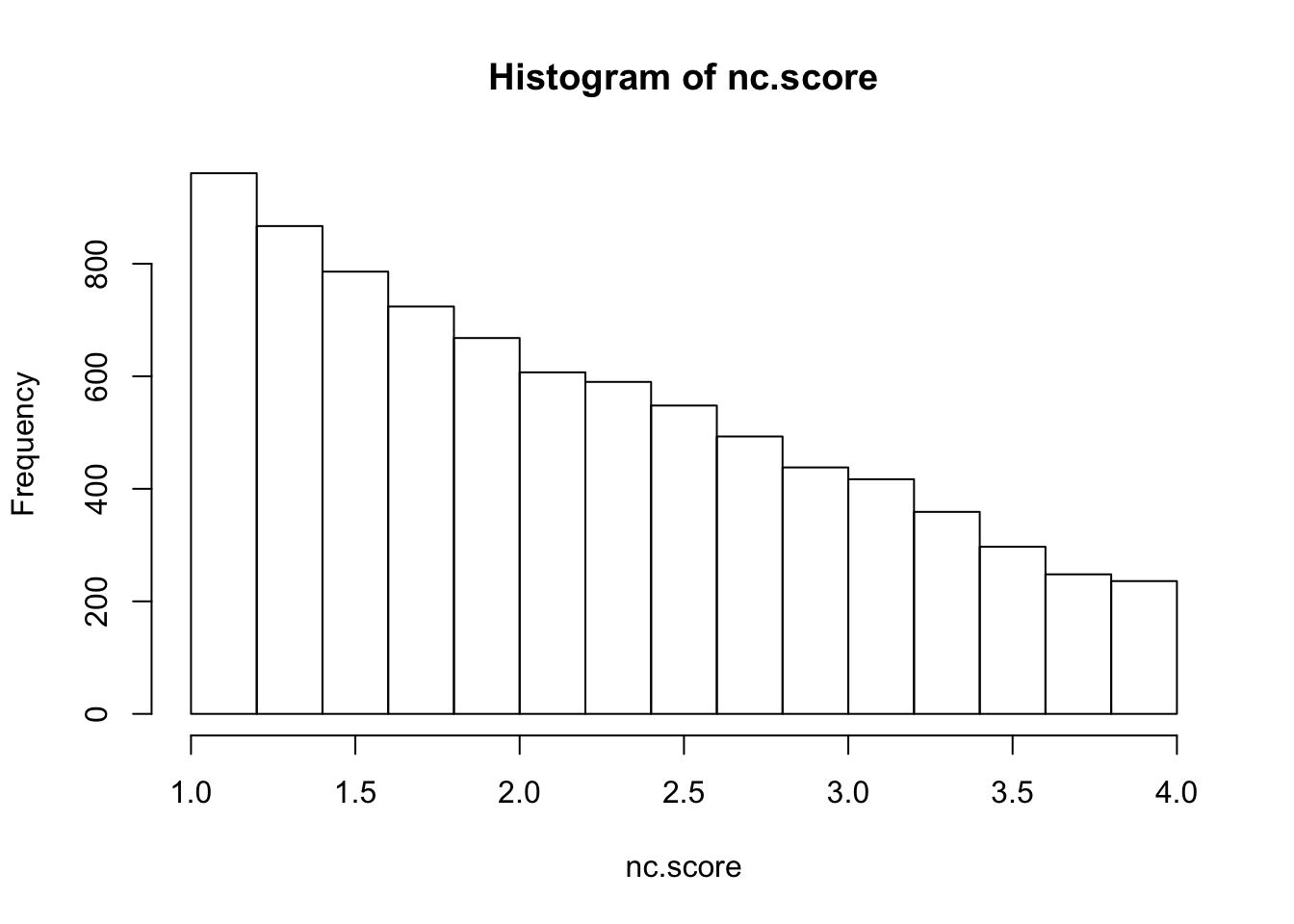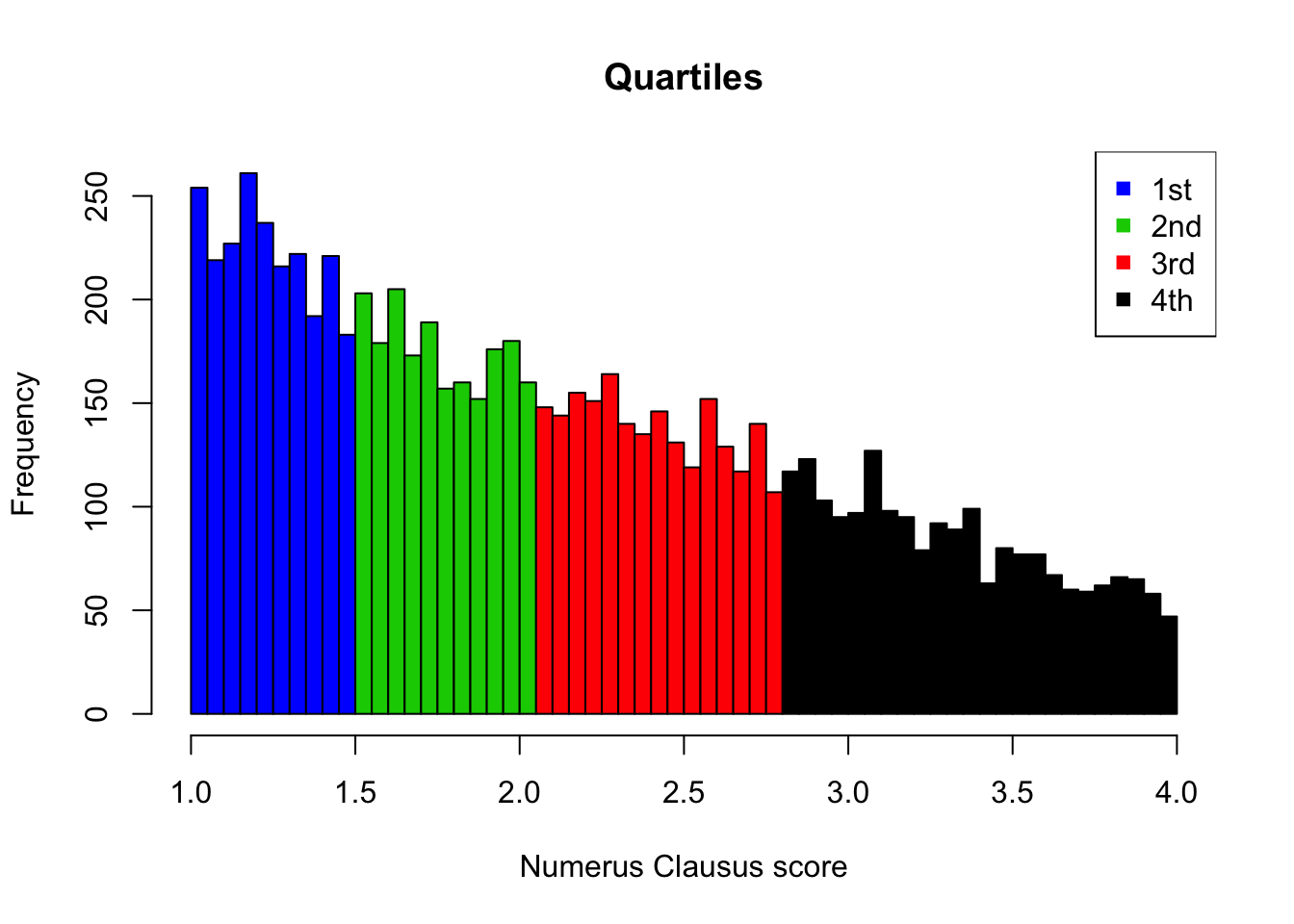Quartiles divide a ranked data set into four equal parts. These three measures are denoted first quartile (denoted by $$Q1$$), second quartile (denoted by $$Q2$$), and third quartile (denoted by $$Q3$$). The second quartile is the same as the median of a data set. The first quartile is the value of the middle term among the observations that are less than the median, and the third quartile is the value of the middle term among the observations that are greater than the median (Mann 2012).Approximately 25% of the values in a ranked data set are less than $$Q1$$ and about 75% are greater than $$Q1$$. The second quartile, $$Q2$$, divides a ranked data set into two equal parts; hence, the second quartile and the median are the same. Approximately 75% of the data values are less than $$Q3$$ and about 25% are greater than $$Q3$$. The difference between the third quartile and the first quartile for a data set is called the interquartile range ($$IQR$$) (Mann 2012).

$IQR = Q3-Q1$

Let us switch to R and test its functionality for computing quantiles/quartiles. We will use the nc.score variable of the studentsdata set to calculate quartiles and the $$IQR$$. The nc.scorevariable corresponds to the Numerus Clausus score of each particular student.

First, we subset the data and plot a histogram to further inspect the variable’s distribution.

students <- read.csv("https://userpage.fu-berlin.de/soga/200/2010_data_sets/students.csv")
nc.score <- students$nc.score hist(nc.score, breaks = 'sturges')To calculate the quartiles for the nc.score variable, we apply the function quantile(). If you call the help() function on quantile(), you see that as default values for the argument probs are set to 0, 0.25, 0.5 and 0.75. Thus, in order to calculate the quartiles for the nc.score variable we just write: quantile(nc.score) ## 0% 25% 50% 75% 100% ## 1.00 1.46 2.04 2.78 4.00 which gives the same result as: quantile(nc.score, probs = c(0, 0.25, 0.5, 0.75, 1)) ## 0% 25% 50% 75% 100% ## 1.00 1.46 2.04 2.78 4.00 Note: Not all statisticians define quartiles in exactly the same way. For a detailed discussion of the different methods for computing quartiles, see the online article “Quartiles in Elementary Statistics” by E. Langford (2006). In addition, you may find the help(quantile) function helpful. In order to calculate the $$IQR$$ for the nc.score variable we either write… nc.score.quart <- quantile(nc.score, names = FALSE) nc.score.quart - nc.score.quart ##  1.32 …or we apply the in-built function IQR(): IQR(nc.score) ##  1.32 We can visualize the partitioning of the nc.score variable into quartiles by plotting a histogram and by adding a couple of additional lines of code. h <- hist(nc.score, breaks = 50, plot = F) cuts <- cut(h$breaks, c(0, nc.score.quart))

plot(h,
col = rep(c("4","4","3","2","1"))[cuts],
main = 'Quartiles',
xlab = 'Numerus Clausus score')

pch = 15)